Function Repository Resource:

# FormatFactorization

Express an integer factorization as it would be seen in a factorization table

Contributed by: Ed Pegg Jr
 ResourceFunction["FormatFactorization"][integer] expresses integer in a factored form as it would be seen in a factorization table.

## Examples

### Basic Examples (1)

Factor various numbers:

 In:=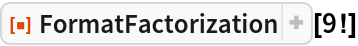Out=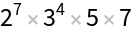In:=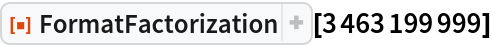Out=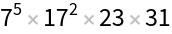In:=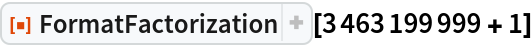Out=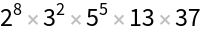In:=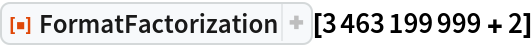Out=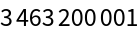### Scope (1)

Make a factorization table:

 In:=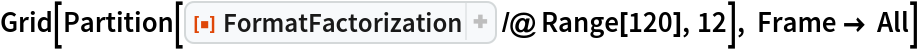Out=## Requirements

Wolfram Language 11.3 (March 2018) or above

## Version History

• 1.0.0 – 27 February 2019